Open In App
Related Articles
• CBSE Class 9 Maths Revision Notes
• CBSE Class 9 Maths Formulas
• NCERT Solutions for Class 9 Maths
• RD Sharma Class 9 Solutions

# Area of Polygons

Area of the Polygon is the area enclosed by the boundary of the polygon. A polygon is a figure formed by joining ‘n’ straight lines such that it forms a closed figure. A polygon has n-sides and there are different types of polygon, such as triangle, square, pentagon, etc. The area of the polygon is found by various methods and different types of polygons have different formulas to calculate its area. For example, suppose we have to find the area of a square (4-sided polygon) then its area is found by the formula, (side)2. Similarly, the area of other polygons is found.

In this article, we will learn about the area of a polygon, here we will learn about the area of a regular polygon, the area of an irregular polygon, related examples, and others in detail.

## What is Area of a Polygon?

We define the area of the polygon as the space by a polygon. Suppose we have a n-sided polygon then the area enclosed by all its n-sides is called the area of that polygon. the area of the polygon is measured in the unit square, i.e. it is measured in m2, cm2, etc.

We can also say that area of the polygon is the space occupied by the polygon in a 2-Dimensional plane. Finding the area of the polygon is very important and we can find the area of the polygon by dividing the polygon into two types. before learning more about the area of a polygon let’s first learn about the type of polygon. The two types of polygons are,

• Regular Polygon
• Irregular Polygon

### Regular Polygon

A polygon with all n-sides equal is called a Regular Polygon. Examples of regular polygons are Equilateral Triangles, Squares, Regular Pentagons, etc. The area of these regular polygons is easily calculated by using various formulas.

### Irregular Polygon

A polygon in which all n-sides are not equal is called an Irregular Polygon. Examples of irregular polygons are Scalene Triangles, Rectangles, etc. Area of an irregular polygon is calculated by using various formulas by dividing these polygons into various known regular polygons and then finding their individual area and then adding them to get the required area of the polygon.

## Area of Polygon Formulas

As we already know the area of polygon is found by using various formulas for both Regular polygons and Irregular polygons. Here are the formulas for some common polygons that we encountered in our daily lives.

## Area of Regular Polygons

A regular polygon is a polygon that has equal sides and equal angles. Thus, the technique to calculate the value of the area of regular polygons is based on the formulas associated with each polygon. Let us have a look at the formulas of some commonly used regular polygons. The table added below shows Regular Polygon and the formulas for calculating their areas.

Regular Polygon

Area Formula

Area of Equilateral Triangle√3(side)2/4
Area of Squareside × side
Area of Regular Pentagon(5/2) (side) × (apothem)

Now we see that the formula for area is different for different types of polygons. Suppose we have to give a common formula to find the area of the regular polygon. Then the formula for the same is,

A polygon having equal sides and equal angles is a regular polygon.

Area of Regular Polygon = (½) × Perimeter × Apothem

Area of Regular Polygon = (½) × (Number of Sides) × (Length of One Side) × (Apothem)

The use of this formula is explained in the example added below,

Example: Find the area of the regular polygon if the perimeter of the polygon is 20 cm and Apothem is 5 cm.

Solution:

Given,

• Perimeter of Polygon = 20 cm
• Length of Apothem = 5 cm

Area of Regular Polygon(A) = (½) × Perimeter × Apothem

A = (½) × 20 cm × 5 cm

A = 50 cm2

Thus, the area of the regular polygon is 50 cm2

## Area of Irregular Polygon

An irregular polygon is a polygon in which all the sides are unequal. To find the area of an irregular polygon we first divide the Irregular Polygon into smaller polygons such as triangles and quadrilateral and then the area of smaller polygons are found and then sum the of all the polygons is found which gives the area of the irregular polygon.

The same can be explained using the example added below: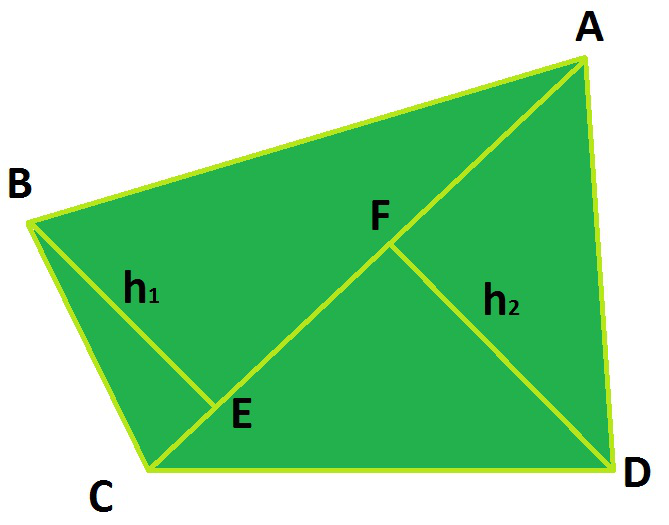Area of Quadrilateral(ABCD) = (Area of Triangle ABC) + (Area of Triangle ACD)

In the above figure, the diagonal AC will act as a common base of the two triangles ABC and ADC with heights h1 and h2 respectively.

Area of Quadrilateral (ABCD) = Area of Triangle (ABC) + Area of Triangle (ACD)

Area of Quadrilateral (ABCD) = (1/2) × AC × h1 + (1/2) × AC × h2

Area of Quadrilateral (ABCD) = (1/2) × AC × (h1 + h2)

Thus, the area of the regular polygon is easily found.

## Area of Polygons with Coordinates

If the coordinates of any polygon are given then its area is calculated using the steps discussed below.

Step 1: First find the distance of all the sides of the polygon, using the distance formula as,

D = √{(x2 – x1)2 + (y2 – y1)2}

Step 2: Finding all the sides of the polygon we first check whether the polygon is a regular polygon or not.

Step 3: Now, if the polygon is a regular polygon then use the formula,

Area of Regular Polygon = (Number of Sides × Length of One Side × Apothem)/2

Step 4: If the polygon is an irregular polygon then its area is calculated by dividing the polygon into smaller polygons then the area of the smaller polygon is found using the known formula. And then the sum is found which gives the area of the irregular polygon.

Example: Find the area of the polygon with coordinates A(1, 1), B(4, 1), C(1, 3), and D(4, 3)

Solution:

AB = √{(3)2 + (0)2} = 3

BC = √{(3)2 + (2)2} = √13

CD = √{(3)2 + (0)2} = 3

DA = √{(3)2 + (2)2} = √13

Here we see that AB = BC and CD = DA. Hence, ABCD is a rectangle

Therefore Area of Rectangle ABCD is 3√13

## Difference Between Perimeter and Area of Polygons

Polygon is a closed figure formed by joining n-sides. Then its area is the space occupied by the boundary of the polygon. The difference between the perimeter and the area of the polygon is discussed in the table added below:

Perimeter of Polygon

Area of Polygon

Perimeter of a polygon is the sum of all the sides of the polygon. It is the length of all the boundaries of the polygon.Area of the polygon is the space occupied by the boundary of the polygon or the total area enclosed by the polygon.
It is measured in units of length, i.e. it is measured in m, cm, etc.It is measured in units of area, i.e. it is measured in m2, cm2,, etc.

The formula to calculate the perimeter of an n-sided polygon is,

Perimeter of Polygon = Length of First Side + Length of Second Side + ….. + Length of N side

The formula to calculate the area of the polygon is,

Area of Polygon = (½) × Perimeter × Apothem

## Area of Polygon Examples

Example 1: Find the area of the given polygon.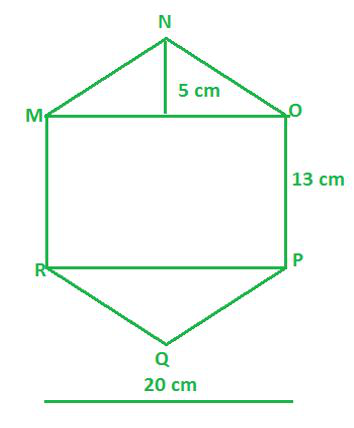Solution:

We are given the above figure which is a Hexagon and we are supposed to find out the area of the above figure MNOPQR.

Area of the figure MNOPQR = Area of triangle MNO + Area of triangle PQR + Area of rectangle MOPR

Area of the figure MNOPQR = (1/2) × MO × NK +(1/2) × PR × QL + PO × MO

Area of the figure MNOPQR = (1/2) × 20 × 5 + (1/2) x 20 × 5 + 13 × 20

Area of the figure MNOPQR = 10 × 5 + 10 × 5 + 260

Area of the figure MNOPQR = 50 cm2+ 50 cm2 + 260 cm2

Area of the figure MNOPQR = 360 cm2

Hence, the area of the given Hexagon MNOPQR is 360 cm2.

Example 2: Find the area of the given polygon.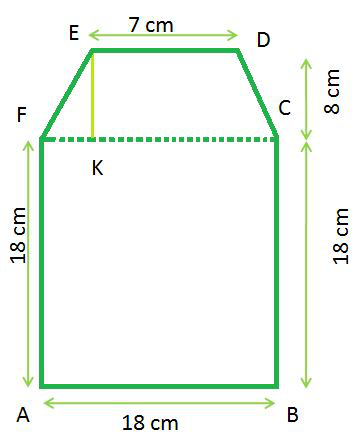Solution:

We are given the above figure which is a Hexagon and we are supposed to find out the area of the above figure ABCDEF.

Area of the figure ABCDEF = Area of Trapezium DEFC + Area of Square ABCF

Area of the figure ABCDEF = (1/2) × (ED+FC) × EK + (AF)2

Area of the figure ABCDEF = (1/2) × (7 + 18) × 8 + (18)2

Area of the figure ABCDEF = 4 × 25 + 18 × 18

Area of the figure ABCDEF = 100 cm2 + 324 cm2

Area of the figure ABCDEF = 424 cm2

Hence, the area of the given Hexagon ABCDEF is 424 cm2

Example 3: Find the area of the given polygon.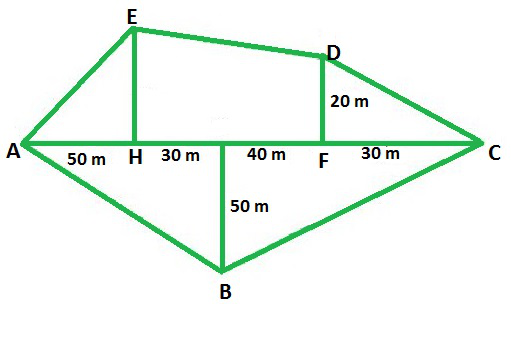Solution:

We are given the above figure which is a Pentagon and we are supposed to find out the area of the above figure ABCDE.

Area of the figure ABCDE = Area of triangle AHE + Area of Trapezium DEHF + Area of triangle DFC + Area of Triangle ABC

Area of the figure ABCDE = (1/2) × AH × HE + (1/2) × (EH+DF) × HF + (1/2)× FC × DF + (1/2) × AC × GB

Area of the figure ABCDE = (1/2) × 50 × 30 + (1/2) × (30+20) × 70 + (1/2) × 30 × 20 + (1/2) × 150 × 50

Area of the figure ABCDE = 25 × 30 + 50 × 35 + 15 × 20 + 150 × 25

Area of the figure ABCDE = 750 m2 + 1750 m2+ 300 m2+ 3750 m2

Area of the figure ABCDE = 6550 m2

Hence, the area of the given Pentagon ABCDE is 6550 m2.

Example 4: Find the area of the given polygon, where the length of the diagonal AC is 18 cm.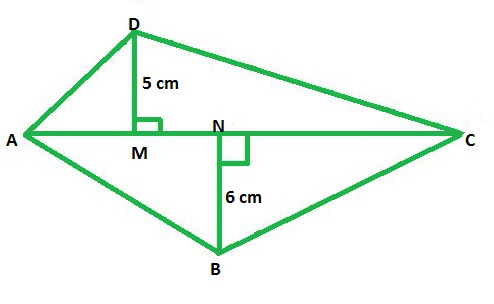Solution:

We are given the above figure which is a quadrilateral, and we are supposed to find out the area of the above figure ABCD.

Area of the quadrilateral ABCD = Area of triangle ABC + Area of triangle ADC

Area of the quadrilateral ABCD = (1/2) × AC × DM + (1/2) × AC × BN

Area of the quadrilateral ABCD = (1/2) × AC × (BN+DM)

Area of the quadrilateral ABCD = (1/2) × 18 × (6+5)

Area of the quadrilateral ABCD = 9 × 11 cm2

Area of the quadrilateral ABCD = 99 cm2

Hence, the area of the given quadrilateral is 99 cm2.

Example 5: Find the area of the given polygon.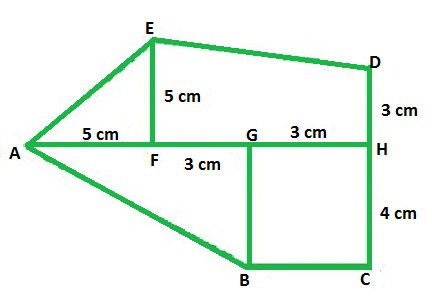Solution:

We are given the above figure which is a Pentagon and we are supposed to find out the area of the above figure ABCDE.

Area of the figure ABCDE = Area of triangle AGB + Area of Rectangle BCHG + Area of triangle AFE + Area of Trapezium DEFH

Area of the figure ABCDE = (1/2) × AG × BG + BG × GH + (1/2) × AF × FE + (1/2) × (DH+EF) × FH

Area of the figure ABCDE = (1/2) × 8 × 4 + 4 × 3 + (1/2) × 5 × 5 + (1/2) × (3+5) × 6

Area of the figure ABCDE = 4 × 4 + 4 × 3 + 2.5 × 5 + 3 × 8 cm2

Area of the figure ABCDE = 16 + 12 + 12.5 + 24 cm2

Area of the figure ABCDE = 64.5 cm2

Hence, the area of the given Pentagon ABCDE is 64.5 cm2.

Example 6: Find the area of the given polygon.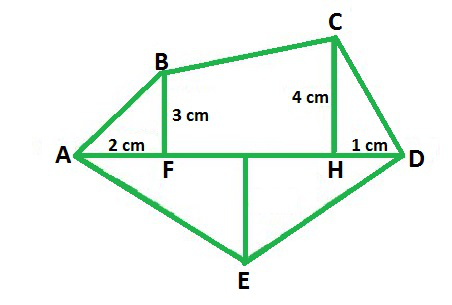Solution:

We are given the above figure which is a Pentagon, and we are supposed to find out the area of the above figure ABCDE.

Area of the figure ABCDE = Area of triangle ADE + Area of triangle CHD + Area of Trapezium BCHF + Area of triangle ABF

Area of the figure ABCDE = (1/2) *AD*GE + (1/2) *HD*CH + (1/2) *(CH+BF)*FH + (1/2) *AF*BF

Area of the figure ABCDE = (1/2) × 7 × 4 + (1/2) × 1 × 4 + (1/2) × (4+3) × 4 + (1/2) × 2 × 3 cm2

Area of the figure ABCDE = 2 × 7 + 2 + 7 × 2 + 1 × 3 cm2

Area of the figure ABCDE = 14 + 2 + 14 + 3 cm2

Area of the figure ABCDE = 33 cm2

Hence, the area of the given Pentagon ABCDE is 33 cm2.

## Practice Questions on Area of Polygons

Q1: Find the Area of an Equilateral Triangle of side 6cm.

Q2: Find the Area of a rectangular park whose length is 10 m and breadth is 30 m.

Q3: What is the Area of Square whose diagonal is 6 cm.

Q4: What is the Area of a Rhombus whose diagonals measures 12 cm and 16 cm?

Q5: What is the Area of a Trapezium whose Parallel sides measures 6 and 12 cm and altitude is 8 cm?

## FAQs on Area of Polygons

### 1. What is Area of Polygon?

Area of the polygon is defined as the area enclosed by the boundries of any polygon. It is the area insides the perimeter of the polygon and is measured in unit square, i.e. it is measured in m2, cm2, etc.

### 2. How to Find the Area of Polygons?

Area of polygon is found by dividing the polygon into two types. Suppose we have to find the area of the regular polygon then its area is calculated using the formula,

Area of Regular Polygon =(½) × Perimeter × Apothem

In case of irregular polygon we find its area by dividing the given polygon in smaller different polygon whose area can be easily calculated and the add all the areas to find the required area.

### 3. What is Area of Polygon with n Sides?

The area of the polygon that has n equal sides is calculated uisng the formula,

Area = [(L2 n)/4 tan(180/n)]

where,

• L is the Length of One Side of Polygon
• n is the Number of Sides of Polygon

### 4. How to Find the Area of Regular Polygons?

The area of the Regular Polygon is easily found using the formula,

Area of Regular Polygon =(½) × Number of Sides × Length of One Side × Apothem

### 5. How to Find the Area of Irregular Polygons?

To find the Area of the irregular polygon we follow the steps discussed below,

Step 1: Divide the given irregular polygon into different polygons for which finding area is easy.

Step 2: Find the area of the individual polygons using appropriate formulas.

Step 3: Find the sum of all the individual areas to find the area of required Irregular Polygon.

### 6. How do you find Area of Polygons with Vertices?

Finding the area of the polygon only if its vertices are given is quite tricky. To calculate the area of the polygon when only its vertices are given follow the following steps.

Step 1: Find the length of all the sides of the polygon using the Distance Formula.

Step 2: Compare the length of all the sides to check whether it is a regular polygon or an irregular polygon.

Step 3: If it is a regular polygon find its area by using the Area of Regular Polygon Formula.

Step 4: If it is an irregular polygon divide the polygon into smaller polygon to get their area easily and then find the sum of all the smaller polygon to get the area of the required polygon.